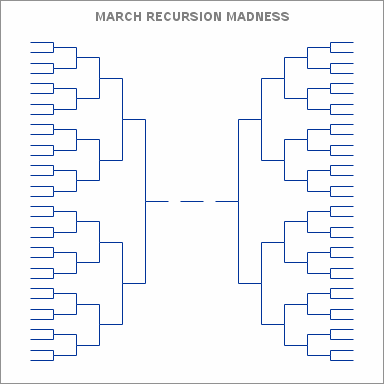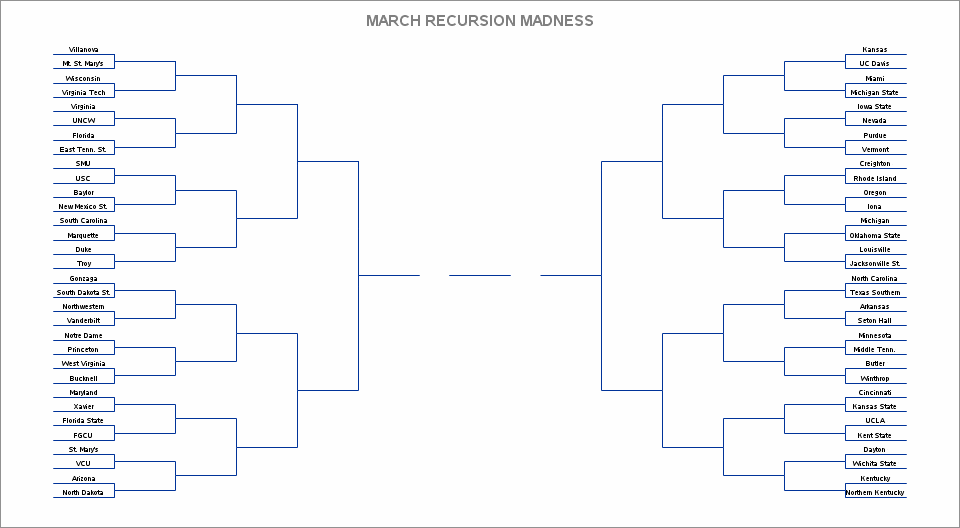## Fun With SAS ODS Graphics: March Recursion Madness (Plotting a 64-Team NCAA Tournament Bracket)This weekend is Selection Sunday, when pairings will be announced for this year's NCAA Men's Basketball Tournament, aka March Madness. Called "America's favorite binary tree", the 64-team NCAA tourney brackets that present the match-ups have even found their way into Ivy League intro-to-CS lecture material. Didn't see an algorithm for drawing one, however, so thought I'd take a stab at coding a short program to create a basic bracket (above animated GIF) using PROC FCMP recursion and an ODS Graphics vector plot. Madness, indeed! 🙂

``````* Fun w/ODS Graphics - NCAA Bracket via FCMP recursion and ODS Graphics step plot;

proc fcmp outlib=work.userfuncs.ncaabracket;      /* Generate bracket points recursively */
function ncaabracket(level, x, y);
static dir;                                       /* Direction: -1/+1=left/right */
if level=6 then dir=x;
x1=x; y1=y; x2=x+dir; y2=y;                       /* Draw horizontal line */
call execute('x1='||x1||'; y1='||y1||'; x2='||x2||'; y2='||y2||'; output;');
if level^=1 then do;
x1=x+dir; y1=y; x2=x+dir; y2=y+2**(level-2);    /* Draw vertical line up */
call execute('x1='||x1||'; y1='||y1||'; x2='||x2||'; y2='||y2||'; output;');
a=ncaabracket(level-1,x2,y2);                   /* Add node up */
x1=x+dir; y1=y; x2=x+dir; y2=y-2**(level-2);    /* Draw vertical line down */
call execute('x1='||x1||'; y1='||y1||'; x2='||x2||'; y2='||y2||'; output;');
rc=ncaabracket(level-1,x2,y2);                  /* Add node down */
end;
return(0);
endfunc;

options cmplib=work.userfuncs;
data _null_;
call execute('data bracket;');                    /* Generate dataset of bracket points */
call execute('x1=-.5; y1=0; x2=.5; y2=0; output;'); /* Generate line for tourney winner */
rc=ncaabracket(6, -1, 0);                         /* Generate points for left side */
rc=ncaabracket(6, 1, 0);                          /* Generate points for right side */

data bracketlinesegments;                         /* One obs per line segment */
set bracket;                                      /* Segments are ordered by creation sequence */
linesegment+1;

proc sql;                                         /* Create "frames" of cumulative line segments */
create table bracketframes as                     /* Will be used to create animated GIF */
select t1.linesegment as frame, t2.*
from bracketlinesegments t1, bracketlinesegments t2
where t1.linesegment>=t2.linesegment order by t1.linesegment, t2.linesegment;

data bracketframes;                               /* Add frame # and title to data */
set bracketframes; by frame;
if first.frame then do; Txt1=put(frame,z3.); xTxt1=0; yTxt1=-32; end;
/* Animated GIF set-up */
options papersize=('4 in', '4 in') printerpath=gif animation=start
nodate nonumber animloop=YES animduration=1.5 NOANIMOVERLAY;
ods graphics / width=4in height=4in imagefmt=GIF;
/* Use vector plot to generate full bracket */
proc sgplot data=bracketframes noborder noautolegend;
where frame=251;                                 /* Just the final frame for 1.5 seconds */
textplot x=xTxt1 y=yTxt1 text=Txt1 / position=top textattrs=(size=18pt color=white) contributeoffsets=none;
vector x=x2 y=y2 / xorigin=x1 yorigin=y1 noarrowheads;
xaxis display=none min=-7 max=7;
yaxis display=none min=-32 max=32;

options animduration=.04 nobyline;               /* Use vector plot to generate 251 incremental brackets */
proc sgplot data=bracketframes noborder noautolegend;
by frame;                                        /* Each frame for .04 seconds */
title color=grey "MARCH RECURSION MADNESS";      /* Add line segment running counter */
text x=xTxt1 y=yTxt1 text=Txt1 / position=top textattrs=(size=18pt color=grey) contributeoffsets=none;
vector x=x2 y=y2 / xorigin=x1 yorigin=y1 noarrowheads;
xaxis display=none min=-7 max=7;
yaxis display=none min=-32 max=32;

options printerpath=gif animation=stop;
run;
ods printer close;``````

2 REPLIES 2Jay54
Meteorite | Level 14

## Re: Fun With SAS ODS Graphics: March Recursion Madness (Plotting a 64-Team NCAA Tournament Bracket)

Awesome, Ted.  As usual, a creative "out-of-the-box" application of SGPLOT.## Re: Fun With SAS ODS Graphics: March Recursion Madness (Plotting a 64-Team NCAA Tournament Bracket)

UPDATE: With the First Four games concluded, the tournament's first round teams are finalized, so the revised code below adds team names (greatly reduced-size animated GIF created from SAS original using compressor.io attached). Btw, the Championship is April 3rd, so if you're SGF 2017-bound, you can catch the game with die-hard fans Monday night at ESPN Club (just across the way from Swan/Dolphin).

REVISED CODE

``````* Fun w/ODS Graphics - NCAA Bracket via FCMP recursion and ODS Graphics step plot;

proc fcmp outlib=work.userfuncs.ncaabracket;      /* Generate bracket points recursively */
function ncaabracket(level, x, y);
static dir;                                       /* Direction: -1/+1=left/right */
if level=6 then dir=x;
x1=x; y1=y; x2=x+dir; y2=y;                       /* Draw horizontal line */
if level=1 then do;
call execute('x1='||x1||'; y1='||y1||'; x2='||x2||'; y2='||y2||'; output;');
call execute('infile pairings; input; team=scan(_infile_,1, "("); xtxt1='||(x1+x2)/2||'; ytxt1='||y||'; output; team=""; x1txt=.; y1txt=.;');
end;
else do;
call execute('x1='||x1||'; y1='||y1||'; x2='||x2||'; y2='||y2||'; output;');
x1=x+dir; y1=y; x2=x+dir; y2=y+2**(level-2);    /* Draw vertical line up */
call execute('x1='||x1||'; y1='||y1||'; x2='||x2||'; y2='||y2||'; output;');
a=ncaabracket(level-1,x2,y2);                   /* Add node up */
x1=x+dir; y1=y; x2=x+dir; y2=y-2**(level-2);    /* Draw vertical line down */
call execute('x1='||x1||'; y1='||y1||'; x2='||x2||'; y2='||y2||'; output;');
rc=ncaabracket(level-1,x2,y2);                  /* Add node down */
end;
return(0);
endfunc;

options cmplib=work.userfuncs;
data _null_;
* Pairings from ncaa.com/interactive-bracket/basketball-men/d1 as of 3/15/2017;
call execute('filename pairings "/folders/myfolders/NcaaPairings.txt"; data bracket; length team \$ 30.;');                    /* Generate dataset of bracket points */
call execute('x1=-.5; y1=0; x2=.5; y2=0; output;'); /* Generate line for tourney winner */
rc=ncaabracket(6, -1, 0);                         /* Generate points for left side */
rc=ncaabracket(6, 1, 0);                          /* Generate points for right side */
run;

data bracketlinesegments;                         /* One obs per line segment */
set bracket;                                      /* Segments are ordered by creation sequence */
linesegment+1;

proc sql;                                         /* Create "frames" of cumulative line segments */
create table bracketframes as                     /* Will be used to create animated GIF */
select t1.linesegment as frame, t2.*
from bracketlinesegments t1, bracketlinesegments t2
where t1.linesegment>=t2.linesegment order by t1.linesegment, t2.linesegment;

data bracketframes;                               /* Add frame # and title to data */
set bracketframes; by frame;
/* Animated GIF set-up */
ods _all_ close;
options papersize=('10 in', '5.5 in') printerpath=gif animation=start
nodate nonumber animloop=YES animduration=1.5 noANIMOVERLAY;
ods graphics / width=10in height=5.5in imagefmt=GIF;
/* Use vector plot to generate full bracket */
proc sgplot data=bracketframes noborder noautolegend nowall;
where frame=327;                                 /* Just the final frame for 1.5 seconds */
text x=xTxt1 y=yTxt1 text=Team / position=top textattrs=(size=5pt color=black) contributeoffsets=none;
vector x=x2 y=y2 / xorigin=x1 yorigin=y1 noarrowheads;
xaxis display=none min=-7 max=7;
yaxis display=none min=-32 max=32;

options animduration=.04 nobyline;               /* Use vector plot to generate 251 incremental brackets */
proc sgplot data=bracketframes noborder noautolegend nowall;
by frame;                                        /* Each frame for .04 seconds */
title color=grey "MARCH RECURSION MADNESS";      /* Add line segment running counter */
text x=xTxt1 y=yTxt1 text=Team / position=top textattrs=(size=5pt color=black) contributeoffsets=none;
vector x=x2 y=y2 / xorigin=x1 yorigin=y1 noarrowheads;
xaxis display=none min=-7 max=7;
yaxis display=none min=-32 max=32;

options printerpath=gif animation=stop;
run;
ods printer close;``````

TEAM PAIRINGS (FROM NCAA.COM)

```Villanova
Mt. St. Mary's
Wisconsin
Virginia Tech
Virginia
UNCW
Florida
East Tenn. St.
SMU
USC
Baylor
New Mexico St.
South Carolina
Marquette
Duke
Troy
Gonzaga
South Dakota St.
Northwestern
Vanderbilt
Notre Dame
Princeton
West Virginia
Bucknell
Maryland
Xavier
Florida State
FGCU
St. Mary's (Cal.)
VCU
Arizona
North Dakota
Kansas
UC Davis
Miami (Fla.)
Michigan State
Iowa State
Purdue
Vermont
Creighton
Rhode Island
Oregon
Iona
Michigan
Oklahoma State
Louisville
Jacksonville St.
North Carolina
Texas Southern
Arkansas
Seton Hall
Minnesota
Middle Tenn.
Butler
Winthrop
Cincinnati
Kansas State
UCLA
Kent State
Dayton
Wichita State
Kentucky
Northern Kentucky```Discussion stats
• 2 replies
• 2880 views
• 8 likes
• 2 in conversation